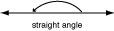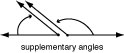## Wednesday, March 28, 2012

Everyone knows that an angle is the space between two lines that intersect.Bamburgh Castle
An Angle can also refer to a member of the Germanic people that invaded England along with the Saxons and Jutes in the fifth century a.d. and merged with them to form the Anglo-Saxon peoples. Here's a photo of Bamburgh Castle, an imposing sandstone castle located on the coast at Bamburgh in Northumberland, England. In 1464, during the Wars of the Roses, Bamburgh became the first castle to succumb to cannon fire, suffering heavy damage. The ruins of the castle were restored and extended in the 18th and 19th centuries, leaving little of the earlier fortifications. Read more.In fishing, angle means "to fish with a hook," from the Old English angel (n.) "angle, hook, fishhook," related to anga "hook," and ang- "to bend" The figurative sense of this word is first recorded from the 1580s.

In Excel Math, students learn about mathematical angles of all sizes. Here's a lesson sheet from Sixth Grade that explains the difference between adjacent, exterior, interior, and corresponding angles.Excel Math Sixth Grade Lesson Sheet

An angle is made up of two rays or line segments that intersect or have the same endpoint. There are many geometric definitions of an angle. Here are a few types of angles:

Acute Angle = an angle that measures less than 90º
Complementary Angles = two angles whose sum equals 90º

Obtuse Angle = an angle that measures more than 90º and less than 180º
Right Angle = a 90º angle (perpendicular lines form right angles where they cross)

Straight Angle = a 180º angleSupplementary Angles = two angles whose sum equals 180º

Exterior Angle = an angle on the outside of two parallel lines that are intersected by another line (r and q are exterior angles on the diagram below)
Interior Angle = an angle on the inside of two parallel lines that are intersected by another line (m and n are interior angles on the diagram)
We see angles of every shape and size in the course of our daily lives. Read about tri-angles in our previous post. It's easy to find angles at school,

at home,
at work,and wherever we go.

See how many angles you and your students can find in each of these pictures. You can even make it a contest to see who can find the most right angles, obtuse angles, and acute angles. What's your angle?

You may also enjoy these articles: